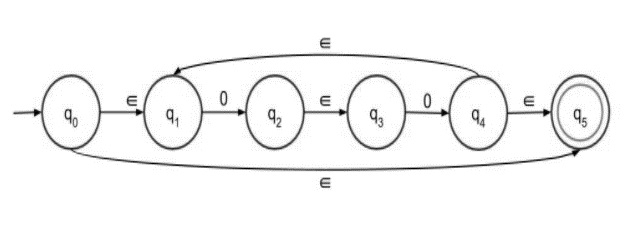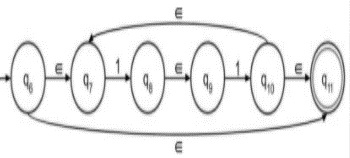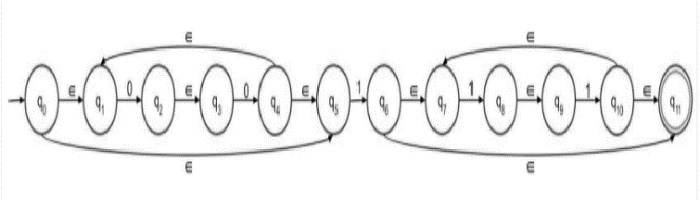# Construct âˆˆ-NFA of Regular Language L = (00)*1(11)*

The ε transitions in Non-deterministic finite automata (NFA) are used to move from one state to another without having any symbol from the input set Σ.

ε-NFA is defined in five tuple representation

{Q, q0, Σ, δ, F}

Where,

• δ − Q × (Σ∪ε)→2Q

• Q − Finite set of states

• Σ − Finite set of the input symbol

• q0 − Initial state

• F − Final state

• δ − Transition function

## NFA without ε transition

NFA also has five states same as DFA, but with different transition function, as shown follows −

$$\delta\colon\:Q\times\:\sum\longrightarrow\:2^{Q}$$

Where,

• Q: Finite set of states

• Σ: Finite set of the input symbol

• q0: Initial state
• F: Final state

• δ: Transition function

Let’s consider the given language L = (00)*1(11)*.

The given language is divided into three parts (00)*, 1 and (11)*. Let’s construct the transition diagram for each part and finally concatenate the three parts to get the final result.

Step 1 − NFA with epsilon for (00)* is as follows −Step 2 − NFA with epsilon for (11)* is as follows −Step 3 − Concatenate first and second part with 1 in between, as follows −